Next: Deconvolution Up: The Wavelet Transform Previous: Hierarchical adaptive filtering

Comparison using a multiresolution quality criterion

It is sometimes useful, as in image restoration where we want to evaluate the quality of the restoration, to compare images with an objective criterion. Very few quantitative parameters can be extracted for that. The correlation between the original image I(i,j) and the restored one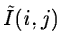gives a classical criterion. The correlation coefficient is: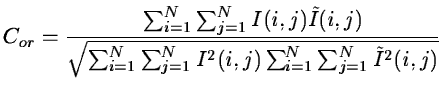(14.95)

The correlation is 1 if the images are identical, and less if some differences exist. Another way to compare two pictures is to determine the mean-square error: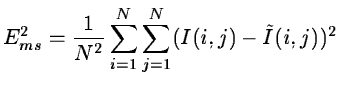(14.96)

Ems2 can be normalized by: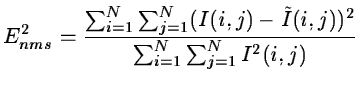(14.97)

The Signal-to-Noise Ratio (SNR) corresponding to the above error is: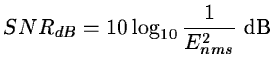(14.98)

These criteria are not sufficient, they give no information on the resulting resolution. A complete criterion must take into account the resolution. For each dyadic scale, we can compute the correlation coefficient and the quadratic error between the wavelet transforms of the original and the restored images. Hence, we can compare, the quality of the restoration for each resolution.

Figures 14.21 and 14.22 show the comparison of three images with a reference image. Data20 is a simulated noisy image, median and wave are the output images after respectively applying a median filter, and a thresholding in the wavelet space. These curves show that the thresholding in the wavelet space is better than the median at all the scales.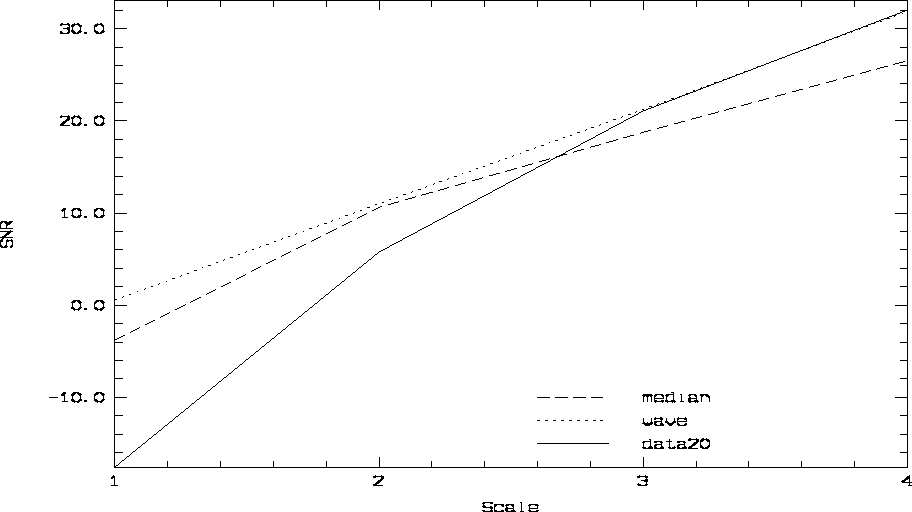Next: Deconvolution Up: The Wavelet Transform Previous: Hierarchical adaptive filtering
Petra Nass
1999-06-15